# Vector - basic operations

There are given points A [-9; -2] B [2; 16] C [16; -2] and D [12; 18]

a. Determine the coordinates of the vectors u=AB v=CD s=DB
b. Calculate the sum of the vectors u + v
c. Calculate difference of vectors u-v
d. Determine the coordinates of the vector w = -7.u

ux =  11
uy =  18
vx =  -4
vy =  20
sx =  10
sy =  2
(u+v)x =  7
(u+v)y =  38
(u-v)x =  15
(u-v)y =  -2
wx =  -77
wy =  -126

### Step-by-step explanation:

$u=\left(2-\left(-9\right);16-\left(-2\right)\right)=\left(11;18\right)$
${u}_{y}=16-\left(-2\right)=18$
$s=\left(12-\left(2\right);18-\left(16\right)\right)=\left(10;2\right)$
${v}_{y}=18-\left(-2\right)=20$
${s}_{x}=12-\left(2\right)=10$
${s}_{y}=18-\left(16\right)=2$
$u+v=\left(11+\left(-4\right);18+\left(20\right)\right)=\left(7;38\right)$
$\left(u+v{\right)}_{y}=18+\left(20\right)=38$
$u-v=\left(11-\left(-4\right);18-\left(20\right)\right)=\left(15;-2\right)$
$\left(u-v{\right)}_{y}=18-\left(20\right)=-2$
$w=-7.u=\left(-7\cdot \left(11\right);-7\cdot \left(18\right)\right)=\left(-77;-126\right)$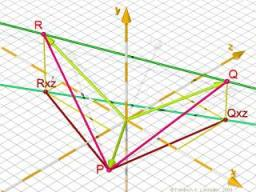Did you find an error or inaccuracy? Feel free to write us. Thank you!Tips to related online calculators
Line slope calculator is helpful for basic calculations in analytic geometry. The coordinates of two points in the plane calculate slope, normal and parametric line equation(s), slope, directional angle, direction vector, the length of the segment, intersections of the coordinate axes, etc.
Our vector sum calculator can add two vectors given by their magnitudes and by included angle.

## Related math problems and questions:

• VectorsVector a has coordinates (8; 10) and vector b has coordinates (0; 17). If the vector c = b - a, what is the magnitude of the vector c?
• Vectors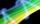For vector w is true: w = 2u-5v. Determine coordinates of vector w if u=(3, -1), v=(12, -10)
• Coordinates of vector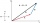Determine the coordinate of a vector u=CD if C(19;-7) and D(-16;-5)
• Coordinates of a centroindLet’s A = [3, 2, 0], B = [1, -2, 4] and C = [1, 1, 1] be 3 points in space. Calculate the coordinates of the centroid of △ABC (the intersection of the medians).
• VectorDetermine coordinates of the vector u=CD if C[19;-7], D[-16,-5].
• Vector sumThe magnitude of the vector u is 12 and the magnitude of the vector v is 8. Angle between vectors is 61°. What is the magnitude of the vector u + v?
• Three points 2The three points A(3, 8), B(6, 2) and C(10, 2). The point D is such that the line DA is perpendicular to AB, and DC is parallel to AB. Calculate the coordinates of D.
• Vector v4Find the vector v4 perpendicular to vectors v1 = (1, 1, 1, -1), v2 = (1, 1, -1, 1) and v3 = (0, 0, 1, 1)
• Two forcesThe two forces F1 = 580N and F2 = 630N, have an angle of 59 degrees. Calculate their resultant force, F.
• Vectors abs sum diff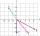The vectors a = (4,2), b = (- 2,1) are given. Calculate: a) |a+b|, b) |a|+|b|, c) |a-b|, d) |a|-|b|.
• Unit vector 2DDetermine coordinates of unit vector to vector AB if A[-6; 8], B[-18; 10].
• Linear independence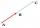Determine if vectors u=(-4; -10) and v=(-2; -7) are linear dependent.
• Space vectors 3DThe vectors u = (1; 3; -4), v = (0; 1; 1) are given. Find the size of these vectors, calculate the angle of the vectors, the distance between the vectors.
• Three points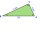Three points K (-3; 2), L (-1; 4), M (3, -4) are given. Find out: (a) whether the triangle KLM is right b) calculate the length of the line to the k side c) write the coordinates of the vector LM d) write the directional form of the KM side e) write the d
• LineStraight-line passing through points A [-3; 22] and B [33; -2]. Determine the total number of points of the line in which both coordinates are positive integers.
• CuboidsTwo separate cuboids with different orientation in space. Determine the angle between them, knowing the direction cosine matrix for each separate cuboid. u1=(0.62955056, 0.094432584, 0.77119944) u2=(0.14484653, 0.9208101, 0.36211633)
• Medians and sidesTriangle ABC in the plane Oxy; are the coordinates of the points: A = 2.7 B = -4.3 C-6-1 Try calculate lengths of all medians and all sides.